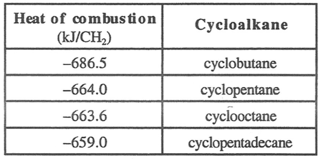Problem: The heat of combustion (per CH 2) of several cycloalkanes is listed below. Based on the data given, which of these cycloalkanes would be considered most stable? a) cyclobutane b) cyclopentane c) cyclooctane d) cyclopentadecane

FREE Expert Solution
83% (179 ratings)
Problem Details

The heat of combustion (per CH 2) of several cycloalkanes is listed below. Based on the data given, which of these cycloalkanes would be considered most stable?

a) cyclobutane

b) cyclopentane

c) cyclooctaneWhat scientific concept do you need to know in order to solve this problem?

Our tutors have indicated that to solve this problem you will need to apply the Alkane Combustion concept. If you need more Alkane Combustion practice, you can also practice Alkane Combustion practice problems .

What is the difficulty of this problem?

Our tutors rated the difficulty of The heat of combustion (per CH 2) of several cycloalkanes is... as medium difficulty.

How long does this problem take to solve?

Our expert Organic tutor, Chris took 2 minutes to solve this problem. You can follow their steps in the video explanation above.

What professor is this problem relevant for?

Based on our data, we think this problem is relevant for Professor Leslie's class at UCM.